Finding AP

Chapter 5 Class 10 Arithmetic Progressions (Term 2)
Concept wise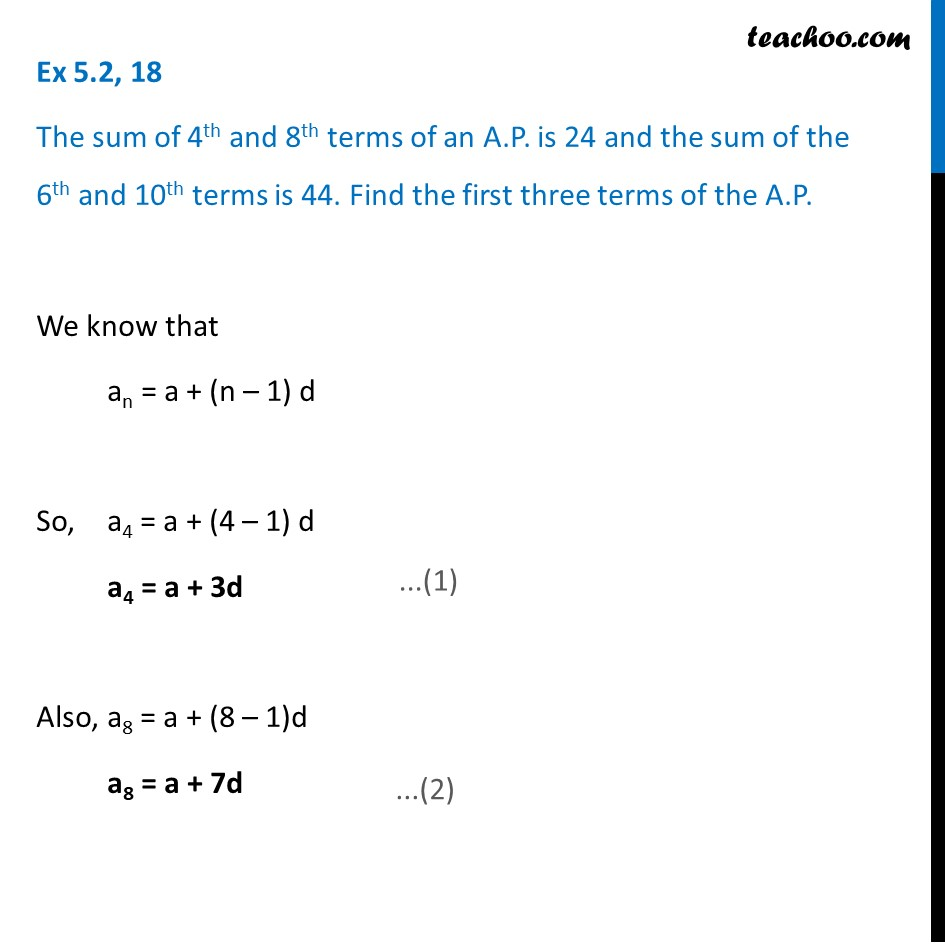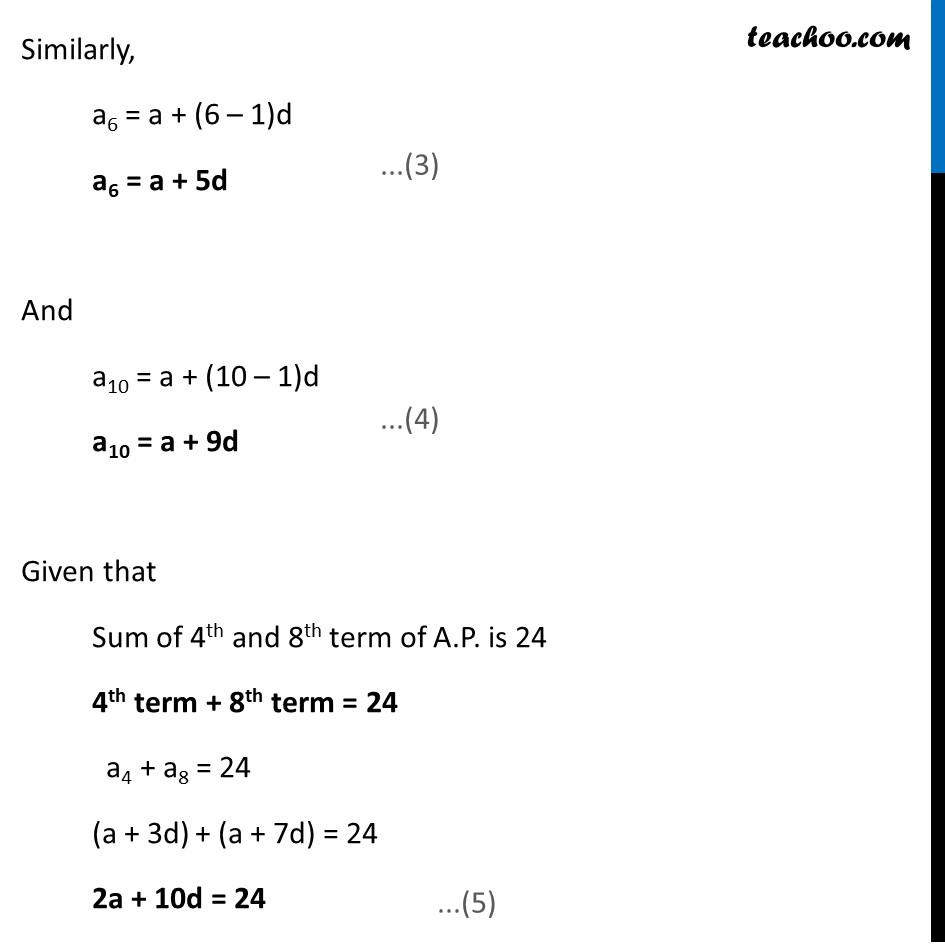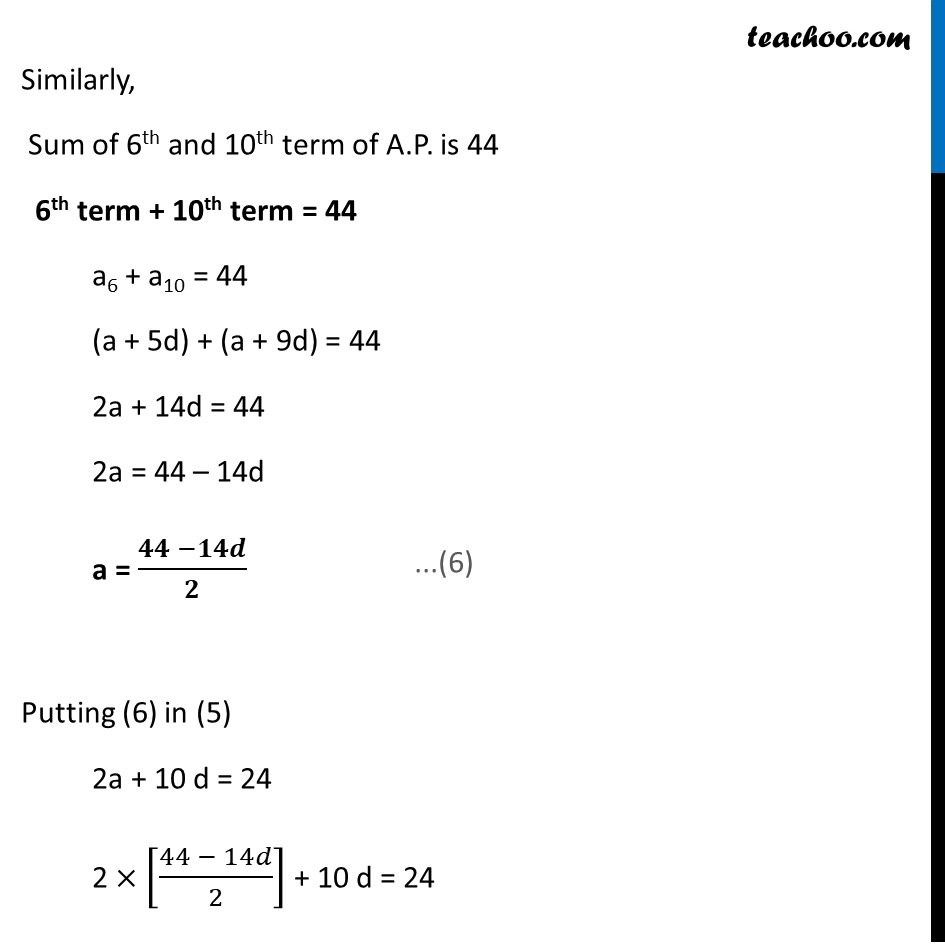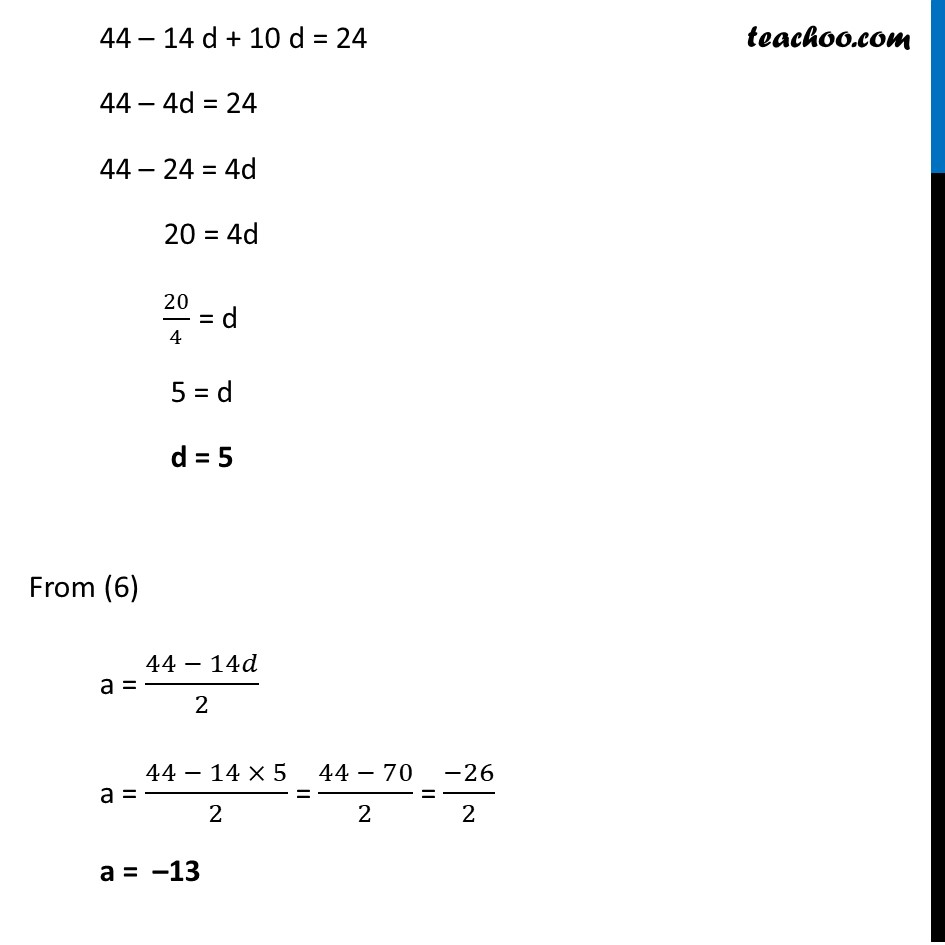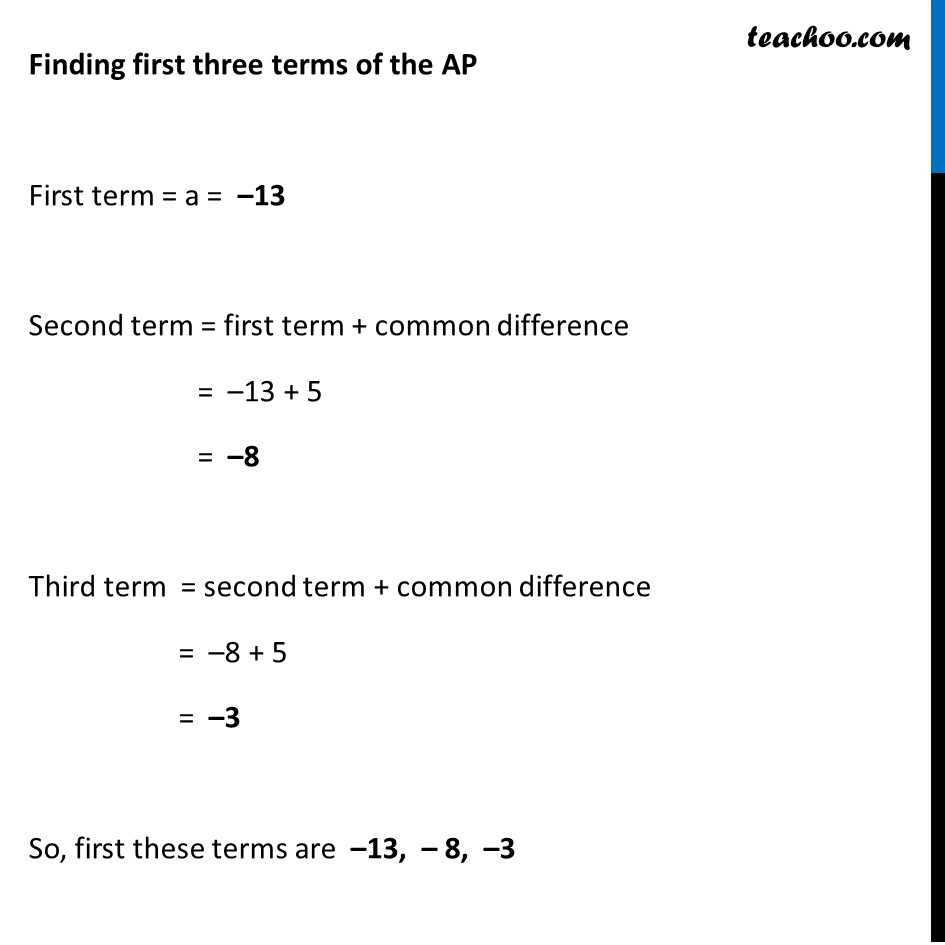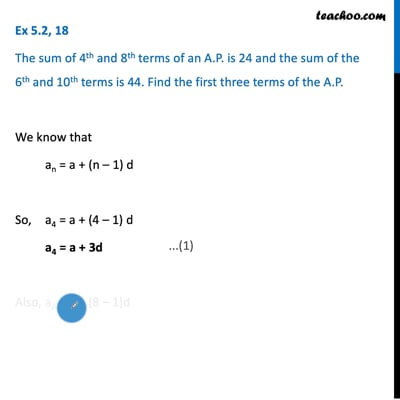This video is only available for Teachoo black users

### Transcript

Ex 5.2, 18 The sum of 4th and 8th terms of an A.P. is 24 and the sum of the 6th and 10th terms is 44. Find the first three terms of the A.P. We know that an = a + (n – 1) d So, a4 = a + (4 – 1) d a4 = a + 3d Also, a8 = a + (8 – 1)d a8 = a + 7d Similarly, a6 = a + (6 – 1)d a6 = a + 5d And a10 = a + (10 – 1)d a10 = a + 9d Given that Sum of 4th and 8th term of A.P. is 24 4th term + 8th term = 24 a4 + a8 = 24 (a + 3d) + (a + 7d) = 24 2a + 10d = 24 Similarly, Sum of 6th and 10th term of A.P. is 44 6th term + 10th term = 44 a6 + a10 = 44 (a + 5d) + (a + 9d) = 44 2a + 14d = 44 2a = 44 – 14d a = (𝟒𝟒 −𝟏𝟒𝒅)/𝟐 Putting (6) in (5) 2a + 10 d = 24 2 × [(44 − 14𝑑)/2] + 10 d = 24 44 – 14 d + 10 d = 24 44 – 4d = 24 44 – 24 = 4d 20 = 4d 20/4 = d 5 = d d = 5 From (6) a = (44 − 14𝑑)/2 a = (44 − 14 × 5)/2 = (44 − 70)/2 = (−26)/2 a = –13 Finding first three terms of the AP First term = a = –13 Second term = first term + common difference = –13 + 5 = –8 Third term = second term + common difference = –8 + 5 = –3 So, first these terms are –13, – 8, –3